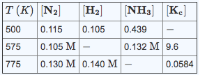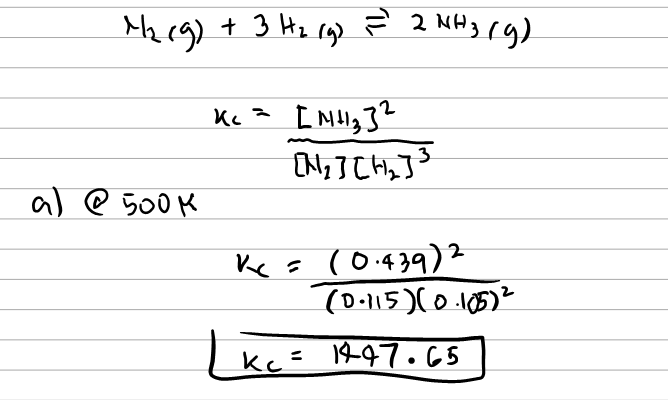Chemistry Practice Problems Equilibrium Expressions Practice Problems Solution: Consider the following reaction N2 (g) + 3H2 (g) ⇌...

🤓 Based on our data, we think this question is relevant for Professor DeSimone's class at UA.

# Solution: Consider the following reaction N2 (g) + 3H2 (g) ⇌ 2NH3 (g) Complete the following table. Assume that all concentrations are equilibrium concentrations in M. Part A Find Kc at 500 K. Part B Find [H2] at 575 K. Part C Find [NH3] at 775 K.

###### Problem

Consider the following reaction

N2 (g) + 3H2 (g) ⇌ 2NH3 (g)

Complete the following table. Assume that all concentrations are equilibrium concentrations in M.Part A

Find Kc at 500 K.

Part B

Find [H2] at 575 K.

Part C

Find [NH3] at 775 K.

###### SolutionView Complete Written Solution

Equilibrium Expressions

Equilibrium Expressions

#### Q. The equilibrium constant, Kc, for the following reaction is 2.01 x 10-2 at 710 K. NH4I (s) ⇌ NH3 (g) + HI (g) Calculate Kc at this temperature for the...

Solved • Thu Aug 30 2018 13:20:59 GMT-0400 (EDT)

Equilibrium Expressions

#### Q. Consider the reaction for the Haber synthesis of ammonia: N2(g) + 3H2 (g) → 2NH3 (g). Which of the following is the correct equilibrium expression? (i...

Solved • Thu Aug 30 2018 13:15:00 GMT-0400 (EDT)

Equilibrium Expressions

#### Q. For A + 2B ⇌ 2C                Kc = 2.15 For 2C ⇌ D                                             Kc = 0.194 Calculate the value of the equilibrium cons...

Solved • Wed Aug 22 2018 13:36:45 GMT-0400 (EDT)

Equilibrium Expressions

#### Q. At 327 K, this reaction has a Kc values of 0.0474. X (g) + 3Y (g) ⇌ 2Z (g) Calculate Kp at 327 K. Note that the pressure is in units of atmosphere (at...

Solved • Wed Aug 22 2018 13:31:06 GMT-0400 (EDT)